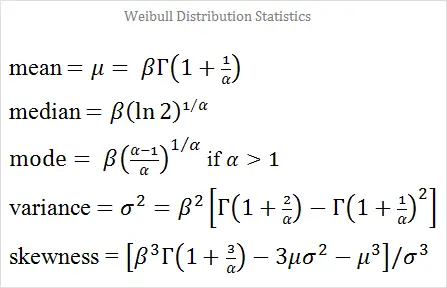# Weibull Random Number Generator

The Weibull distribution with parameters α and β is often used in predicting time to failure and other processes in physics, engineering, and materials science. When you set α = 1, you obtain the exponential distribution; when you set α = 2, you get the Rayleigh distribution, which arises when studying two-dimensional wind speed vectors.

You can generate random variables from a Weibull distribution using the calculator below.

 α =       β =       N = Decimal Precision integer tenths hundredths thousandths Display comma separated vertical

The Weibull probability density function is

PDF(x) = αβxα-1e-(x/β)α    x ∈ [0, ∞)

The mean, median, mode, variance, and skewness of the Weibull distribution are somewhat complicated functions of α and β:When α = 1 (exponential distribution) or α = 2 (Rayleigh distribution), these values can be computed explicitly. For larger values of α, you can use the Weibull distribution calculator to compute the theoretical mean, median, mode, variance, and skewness. You can also use the stats calculator to compute the mean, median, mode, variance, etc. of the numbers generated by this random variable tool.